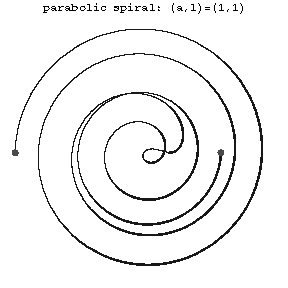# Difference between revisions of "Parabolic spiral"

A transcendental plane curve whose equation in polar coordinates has the form \begin{equation} \rho = a\sqrt{\phi} + l,\quad l>0. \end{equation} To each value of $\phi$ correspond two values of $\sqrt{\phi}$, one positive and one negative.The curve has infinitely many double points and one point of inflection (see Fig.). If $l=0$, then the curve is called the Fermat spiral. The parabolic spiral is related to the so-called algebraic spirals (see Spirals).